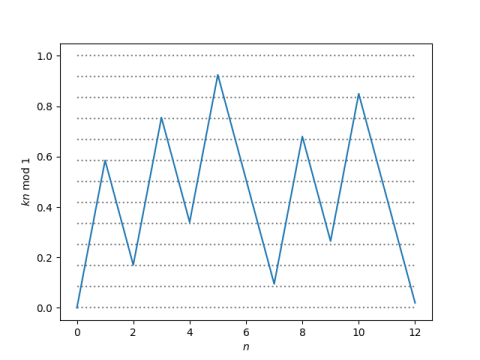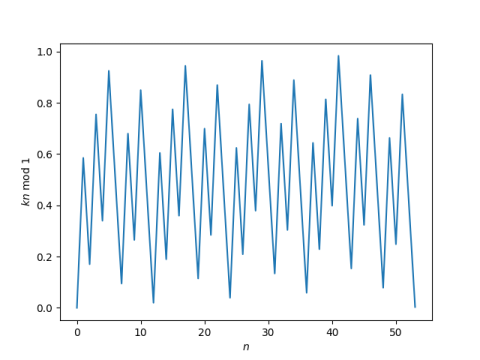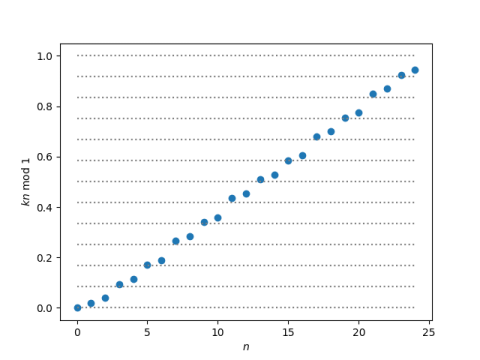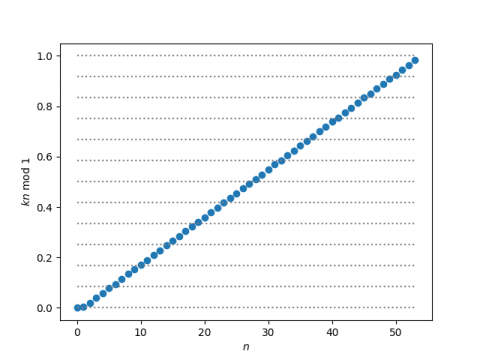# Perfect fifths, octaves, and an ergodic map

In music, a perfect fifth is the interval between two notes whose frequencies are in 3:2 ratio. For example, the interval from an A at 440 Hz and an E at 660 Hz is a perfect fifth.

Going up by 12 perfect fifths is very nearly the same as going up 7 octaves. That is,

(3/2)12 ≈ 27

or in other words,

27/12 ≈ 3/2.

This is why equal temperament tuning works. More on that here.

## 53-note scale

Going back to our first approximation, we can say that (12, 7) is an approximate solution to the equation

(3/2)x = 2y.

One could naturally ask whether there are better approximate solutions. One such solution, dating back to 40 BC, came from the Chinese scholar King Fang . He discovered that (53, 31) is a substantially more accurate solution than (12, 7), between 6 and 7 times better.

```    >>> 1.5**12/2**7
1.0136432647705078
>>> 1.5**53/2**31
1.0020903140410862
```

In other words, going up 53 perfect fifths is nearly the same as going up 31 octaves. You could divide the octave into 53 parts using perfect fifths; we will demonstrate this visually below. However, we’re moving more the realm of number theory than practical music theory at this point.

## Powers of 2 and 3

We could change our problem slightly, making it mathematically simpler though less obviously related to music, by putting all our powers of 2 on one side and looking for powers of 3 that approximately equal powers of two. That is, we could look at approximate solutions to

3x = 2y.

where now x and y have different meanings; our new y is our old y plus our old x. No power of 3 will ever equal a power of 2, but you can find powers of 3 close to powers of 2, making the ratio as small as you’d like.

## Ergodic map

Taking the logarithm of both sides in base 2, we recast the problem as looking for integers n such that

log2(3) n

is approximately an integer.

If we define k = log2(3), we are looking for n such that kn mod 1, the integer part of kn, is near 0.

The map

nkn mod 1

is ergodic because k is irrational, and so its image in the unit interval is dense as n goes to infinity. This means we can find solutions as close we’d like to 0. It also means we can find solutions as close as we’d like to any other number in the interval.

Here’s a plot of our map for n up to 12.Note that the range of the map falls very nearly on the 12 evenly-spaced horizontal lines. This corresponds to the circle of fifths filling in the 12 notes of the chromatic scale.

Now let’s keep going for n up to 53.Look closely at the bottom of the plot. The graph gets close to 0 at 12, and it gets even closer to 0 at 53. The value of kn mod 1 doesn’t get smaller than the value at n = 53 until n = 359 where the value is about twice as small.

When we go up by 12 perfect fifths, we don’t end up exactly on the note we started on; 12 fifths is a little more than 7 octaves. If we keep going up in fifths we fill in notes a little higher than the original chromatic scale. Here’s a plot for n up to 24, with the values sorted so we can focus on the range rather than the ups and downs of filling in the range.Every note in the original chromatic scale now has a counterpart that’s somewhere around 25 cents sharp.

If we keep going to n = 53, we fill in the octave more evenly. Here’s a plot of the sorted values.## Related posts

 A. L. Leigh Silver. Some Musico-Mathematical Curiosities. The Mathematical Gazette , Feb., 1964, Vol. 48, No. 363 (Feb., 1964), pp. 1-17.

## One thought on “Perfect fifths, octaves, and an ergodic map”

1. Ross Mohan

Our host mentions “practical music theory“. Focusing purely on the first of those three words, I wonder if the various “temperings“ of scales were designed to capture — in the first handful of harmonics — virtually all of the musical power spectral density, and therefore what the listener actually hears.

It could be that the worse approximation is actually better in a limited range of harmonics that we’re likely to hear in live music, and that’s what drove its historical choice over a theoretically more precise approximation?

multiple caveats: I’ve merely skimmed John’s post, am neither musician nor musical theorist, and I don’t know exactly how many harmonics in a typical instrument capture, say, 99% of the power spectral density.

(Apologies for the awkward phrasing; just wanted to put the idea out there for the cognoscenti…)